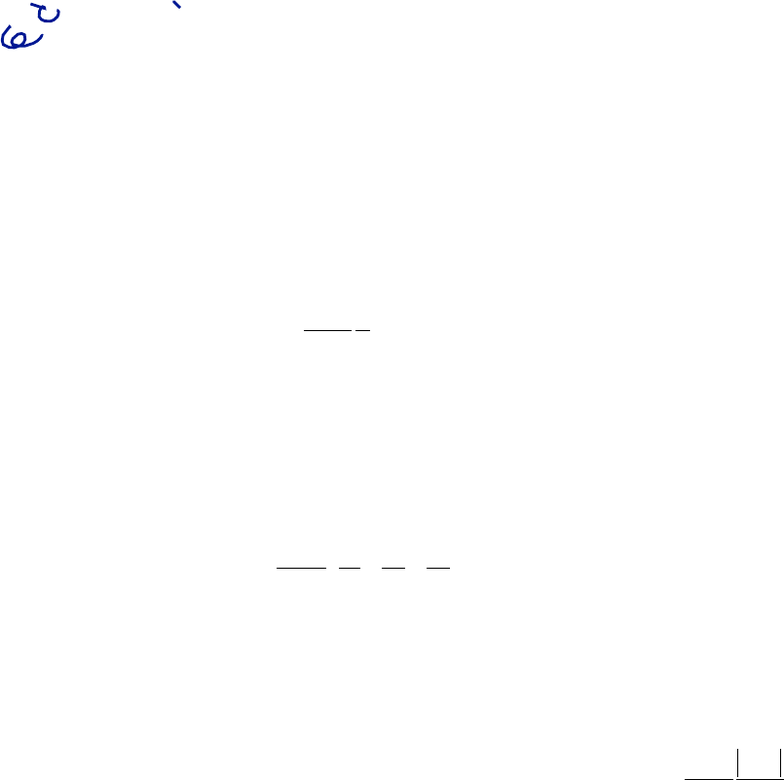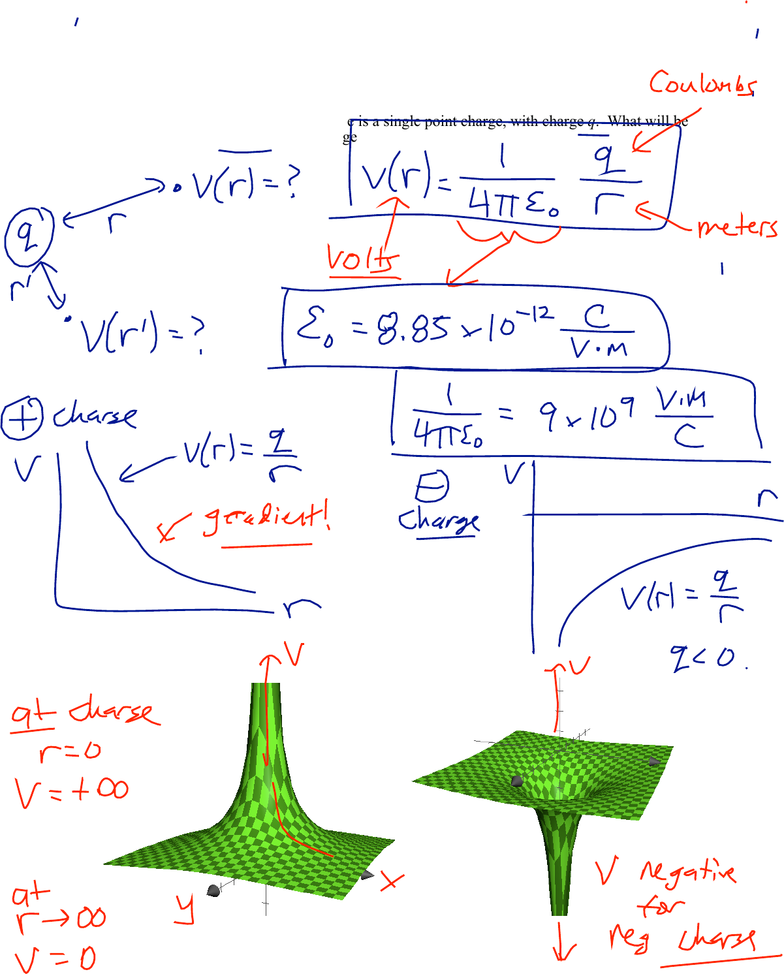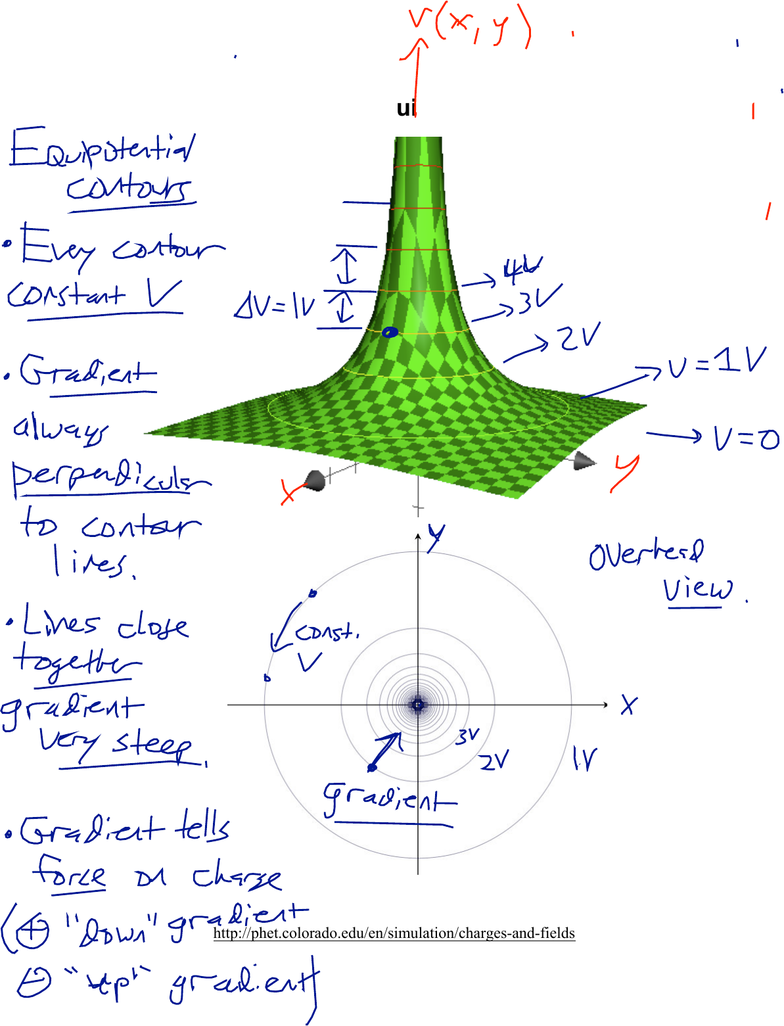Study Guides (400,000)
US (230,000)
Harvard (700)
(20)
Final

# Physical Sciences 3 Lecture Notes - Lecture 2: Electric Potential, Equipotential, Physical ConstantExam

Department
Physical Sciences
Course Code
Physical Sciences 3
Professor
Roxanne Guenette
Study Guide
Final

This preview shows pages 1-2. to view the full 8 pages of the document.Physical Sciences 3: Lecture 1b
January 31, 2019
1
Charges and Potential
A single point charge creates a potential that decreases as 1/r, where r is the distance
from the charge. A positive charge creates a positive potential; a negative charge creates
a negative potential. Expressed in terms of the physical constant
ε
0, the potential is:
V(r)=1
4
πε
0
q
r
Potentials from several charges simply add (this is the principle of superposition):
Vtotal = V1 + V2 + V3 + …
where V1 is the potential due to charge 1, etc. For a bunch of point charges this is:
V=1
4
πε
0
q1
r
1
+q2
r2
+q3
r3
+
From the potential of a point charge, and using our general relationship between force
and potential, we can derive Coulomb’s Law, which says that two point charges will
have a force that acts along a straight line between them. Charges of the same sign will
be repelled; charges of opposite sign will be attracted:
Coulomb’s Law: force has a magnitude of
F=1
4
πε
0
q1q2
r2
Learning objectives: After this lecture, you will be able to…
1. Calculate the electric potential created by a point charge.
2. Use the superposition principle to find the electric potential from several point charges.
3. Draw equipotential contour lines to represent the electric potential of a single charge,
several charges, or other possible scenarios.
4. Estimate the magnitude and direction of the gradient of a field, given contour lines for the
field.
5. Use equipotential contour lines to estimate the magnitude and direction of the
electrostatic force on a charge.
6. Derive and use Coulomb’s Law to find the force exerted by one point charge on another
point charge.
7. Calculate the potential created by a continuous distribution of charge by integrating over
all of the infinitesimal “bits of charge” dq.

Only pages 1-2 are available for preview. Some parts have been intentionally blurred.Physical Sciences 3: Lecture 1b
January 31, 2019
2
The Electric Potential of a Point Charge
Suppose all there is in the universe is a single point charge, with charge q. What will be
the potential created by that charge?
How can we represent this potential?

Unlock to view full version

Only pages 1-2 are available for preview. Some parts have been intentionally blurred.Physical Sciences 3: Lecture 1b
January 31, 2019
3
Mapping the Potential: Equipotential Contour Lines